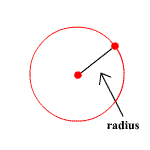A radius of a circle (or sphere) is a line segment with one endpoint at the center, and the other endpoint at the boundary. The word is also used to mean the length of such a segment.All the radii of a particular circle (or sphere) are equal in length. The length of the radius of a circle is equal to

$\frac{C}{2\pi }$ ,

where $C$ is the circumference of the circle, and $\pi$ is pi , an irrational number approximately equal to $3.14$ .

The radius is also used in the calculation of the area of a circle. The formula is

$A=\pi {r}^{2}$ .